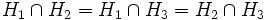# Union of three proper subgroups is the whole group implies they have index two and form a flower arrangement

## Name

This result is often termed Scorza's theorem since it was first proved in a paper by Scorza.

## Statement

Suppose$G$ is a group and$H_1, H_2, H_3$ are proper subgroups of$G$ such that the union is the whole group$G$:$G = H_1 \cup H_2 \cup H_3$

Then each$H_i$ has index two in$G$, and they form a flower arrangement of subgroups:$H_1 \cap H_2 = H_1 \cap H_3 = H_2 \cap H_3$.

Further, this intersection is a normal subgroup of$G$ and the quotient is isomorphic to the Klein four-group.